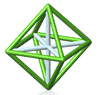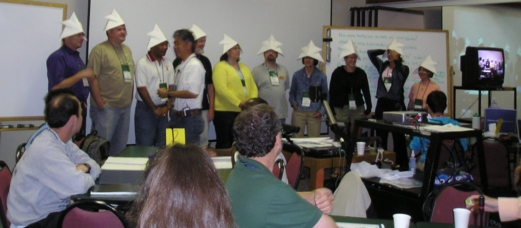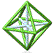Park City Mathematics Institute Secondary School Teacher Program Reflection on Practice Class: Day 10 Akihiko Takahashi

Aki reviewed the results from last week's voting about our posters. Last week, our goal was to choose a typical textbook problem and change it to make it open ended in such a way as that it would elicit a many solutions.

Two tables obtained 14% each 8/54: Table 1 and Table 6. Aki's PowerPoint [download file] contains some comments about the winning posters. The group applauded the participants from the two tables.Aki talked about the three types of open-ended problems:

1. A lesson using problems with multiple solutions (using these once a month in a class would be great)
2. A lesson using problem with multiple solution methods - a typical problem - changed to be open-ended
3. A lesson using an activity called "problem to problem." This is the subject of today's class

How is problem solving different from doing problems as an exercise? The problems given in problem solving should be ones that students don't necessarily know how to solve. In an exercise, students already know how to solve problem and are doing the exercises as practice.

In this third variation of Open Ended Problems, students are given a basic problem. They then create own problem by changing original problem. At the end there is a period of comparing and discussing their problems. This method provides students with opportunities to become active constructors rather than passive receptors. Some teachers may worry whether kids have the potential to do this, but Aki urges that the students can, in fact, create their own problems.

His example involved making squares with toothpicks.
Two squares, seven toothpicks
Three squares, ten toothpicks
Four squares,...
How many toothpicks do you need to make ten squares?

We explored this problem in our groups and came up with a variety of methods:

Method 1: Make a table and use it to come up with a formula: T = 3n + 1. This formula can also be gotten by starting with one toothpick and adding three for each square.

Method 3: T = 2n + (n+1); 2n represents the total toothpicks on the top and bottom. n represents each of the additional toothpicks on right, 1 is the one on the left.

We then worked in our groups to formulate additional problems.

Some of them were:

How many toothpicks are needed to make an n x n square?
What's the area and perimeter of the 10th picture in Aki's scheme?
How many toothpicks are needed to make an n X n X n cube?
What is the pattern when there are 3, 4, 5 sides n-gons - following Aki's example?
Given a fixed # of toothpicks how many squares can you make? What is the least and most # of squares?
Build 10 squares; using different colors, what is the least number of colors so that no two adjacent edges have the same color?
How many toothpicks are needed to make a stairstep of n stairs?
By stacking squares efficiently, what's the least number of toothpicks needed to produce n unit squares?

We discussed these types of problems.

What sort of difficulties might you anticipate with these problems?

• Kids might create problems exactly like the teacher
• Trying to figure out WHAT to do
• The form of a question (givens and goals)
• Do we need an answer?
• Taking into account prior knowledge
• Students, when designing problems, may find themselves over their "mathematical" heads, as complexity can rise quickly.
• Constructing questions can be difficult as they attempt to verbalize them.
• Knowing how to ask the question correctly to avoid ambiguity & confusion is important.
• Students may know what they want, but can't adequately or completely explain.
• Some students may merely focus on the trivial, or change the pattern of the formula, not the mathematics.
• Not many students will think to extend into 3 dimensions but instead just focus on different shapes, arrangements.

• Pattern Building
• Change the "Noun" in the problem
• Or the parameters, algorithm, outputs, inputs, givens, variables

Back to: Class NotesPCMI@MathForum Home || IAS/PCMI Home© 2001 - 2018 Park City Mathematics Institute IAS/Park City Mathematics Institute is an outreach program of the Institute for Advanced Study, 1 Einstein Drive, Princeton, NJ 08540 Send questions or comments to: Suzanne Alejandre and Jim King

With program support provided by Math for America

This material is based upon work supported by the National Science Foundation under DMS-0940733 and DMS-1441467. Any opinions, findings, and conclusions or recommendations expressed in this material are those of the author(s) and do not necessarily reflect the views of the National Science Foundation.